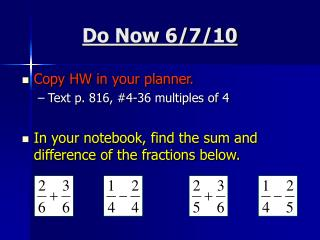DownloadDownload PresentationDo Now 6/7/10

# Do Now 6/7/10

Télécharger la présentation## Do Now 6/7/10

- - - - - - - - - - - - - - - - - - - - - - - - - - - E N D - - - - - - - - - - - - - - - - - - - - - - - - - - -
##### Presentation Transcript

1. Do Now 6/7/10 • Copy HW in your planner. • Text p. 816, #4-36 multiples of 4 • In your notebook, find the sum and difference of the fractions below.

2. Objective • SWBAT add and subtract rational expressions.

3. Section 12.6 “Add and Subtract Rational Expressions” • Adding and subtracting rational expressions is similar to adding and subtracting fractions. • Be sure to simplify your answer. Denominator must be COMMON!!!

4. EXAMPLE 1 Find the sum or difference. Simplify numerator

5. Least Common Denominator • The LCD of two or more rational expressions is the product of the factors of the denominators of the rational expressions with each common factor used only once. (Common factors) x (Uncommon factors)

6. EXAMPLE 2 Find the LCM. (Common factors) x (Uncommon factors) (Common factors) x (Uncommon factors)

7. EXAMPLE 2 Find the LCM. Because c – 2 and 2c +7 cannot be factored, they don’t have any factors in common. The LCM is the product of c – 2 and 2c + 7.

8. Adding and Subtracting with Different Denominators • To add or subtract rational expressions that have different denominators, use the LCD to write equivalent rational expressions that have the same denominator just as you would for numerical fractions.

9. EXAMPLE 3 Find the sum or difference. LCD = 24x³

10. EXAMPLE 4 Find the sum or difference. LCD = 3x(x+2)

11. EXAMPLE 5 Find the sum or difference. LCD = (x+5)(x – 2)(x + 4)

12. Try it out…Find the sum or difference. Homework Text p. 816, #4-36 multiples of 4